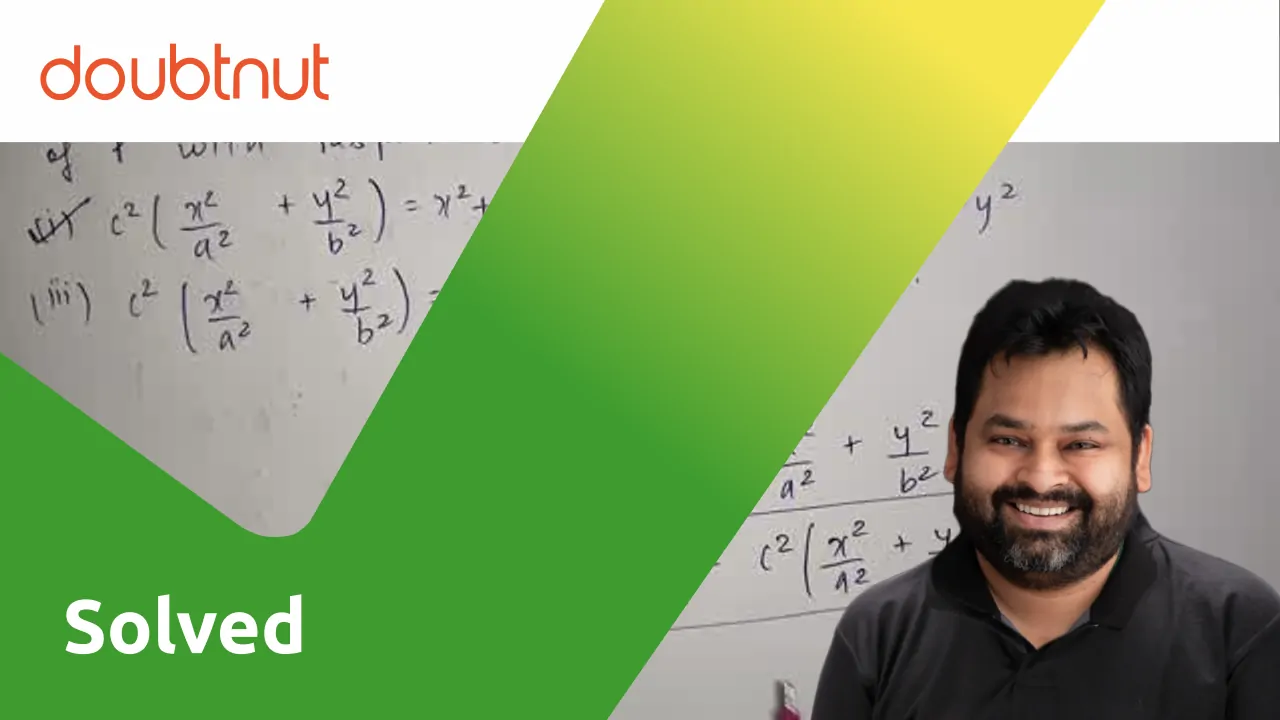Doubtnut App - Get 50 Lakh+ video solutions better than GoogleInstall Now

# P is a point on the circle x2+y2=c2. The locus of the mid-points of chords of contact of P with respect to x2a2+y2b2=1, is

A

c2(x2a2+y2b2)=x2+y2

B

c2(x2a2+y2b2)2=x2+y2

C

c2(x2a2+y2b2)=(x2+y2)2

D

none of these

Text Solution
Verified by Experts

Video Solution